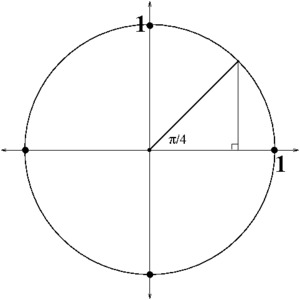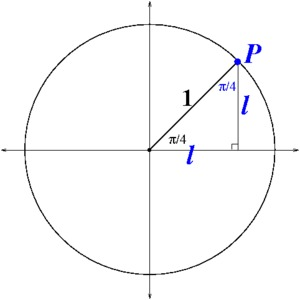# Special Triangles 1

Alignments to Content Standards: F-TF.A.3

Use the unit circle and indicated triangle below to find the exact value of the sine and cosine of the special angle $\pi/4.$Using known facts about the unit circle and isosceles triangles together with the Pythagorean Theorem, we can derive the sine and cosine of special angles, in this case of $\pi/4$. This task can be done as a mini lecture soliciting responses from the students, or as a challenge problem for students to ponder and discuss. It is a very nice connection between geometry and algebra that uses quite simply the symmetry of the triangle. A natural mathematical practice to focus on in this task is SMP 3 - Make a viable argument and critique the reasoning of others. Similar tasks, to derive the exact values of sine and cosine of $\pi/3$ and $\pi/6,$ are in progress. A variant of this task would be to write down the steps in the proof and to ask students to supply the justification.
First we label the vertex of the triangle that lies on the unit circle as point $P$. We know that $P$ has coordinates $(\cos(\pi/4), \sin(\pi/4)).$ Note that the given triangle is an isosceles right triangle with hypotenuse $1,$ so we begin by letting $l$ represent the length of each side of the triangle.Then we have $$l^2 + l^2 = 1^2 \Longrightarrow 2l^2 = 1 \Longrightarrow l^2 = 1/2,$$ which means that $l = \sqrt{1/2},$ which can also be written as $l = \sqrt{2}/{2}.$ It follows that $P = (\sqrt{2}/2,\ \sqrt{2}/2),$ and we conclude that $$\cos (\pi/4) = \frac{\sqrt{2}}{2} \text{ and } \sin (\pi/4) = \frac{\sqrt{2}}{2}.$$ (Note that the last step of rationalizing the denominator is not really necessary, it is just an equivalent way of writing the solution.)